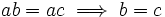# Varying group

(diff) ← Older revision | Latest revision (diff) | Newer revision → (diff)
This is a survey article related to:group
View other survey articles about group

In this article, we explore many variations that are possible in the definition of a group, that yield similar, though slightly weaker or stronger, structures.

To begin with, let us recall the three axioms that describe a group:

• The associativity of the binary operation
• The existence of a neutral element (viz, a left and right multiplicative identity)
• The existence of a multiplicative inverse for every element

The idea behind varying the notion of group would be to remove, or relax, one or more of these assumptions.

## Relaxing the inverse element assumption

### Group without inverses: Monoid

Further information: monoid

A set equipped with an associative binary operation having a neutral element (viz, left and right multiplicative identity) is termed a monoid. Monoids differ from groups in the sense of not necessarily having inverses. Examples of monoids are the set of natural numbers under multiplication, the set of integers under multiplication, the set of integers modulo$n$ under multiplication, the set of all functions from a set to itself (under composition), and more generally, the set of all endomorphisms of a structure.

### Cancellation monoid

Further information: Cancellation monoid

Due to the existence of inverses in a group we can do left and right cancellation. Let's run through the proof quickly:$ab = ac \implies a^{-1}(ab) = a^{-1}(ac) \implies (a^{-1}a)b = (a^{-1}a)c \implies b = c$

A natural question: are there monoids where we can do both left and right cancellation, viz:$ab = ac \implies b = c$

and$ac = bc \implies a = b$

even without assuming the existence of inverses? Actually there do exist such monoids, and such monoids are called cancellation monoids. The following are true:

• Any submonoid of a cancellation monoid is a cancellation monoid. In particular, any submonoid of a group is a cancellation monoid. Thus, the natural numbers under multiplication form a cancellation monoid, because they can be embedded in the group of nonzero rationals under multiplication.
• Any Abelian cancellation monoid can be embedded as a submonoid of an Abelian group. However, the corresponding statement is not true for general cancellation monoids: there are cancellation monoids that cannot be embedded into any group.